## Thursday, April 19, 2012

### Survival Analysis

Let ‘T’ be a random variable time to event. Then the distribution of ‘T’ can be described as follows: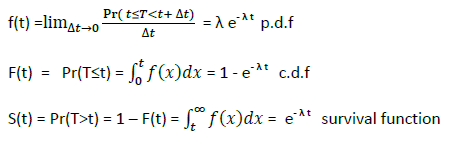The Hazard function is a conditional density function giving the instantaneous risk that an event will occur at time‘t’.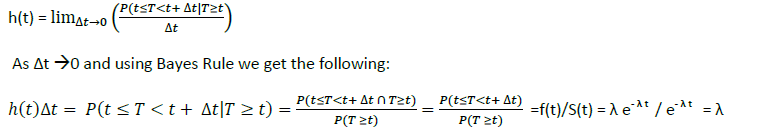Using R, crude plots of f(t), F(t), S(t), and h(t)  for λ = .5 are given below: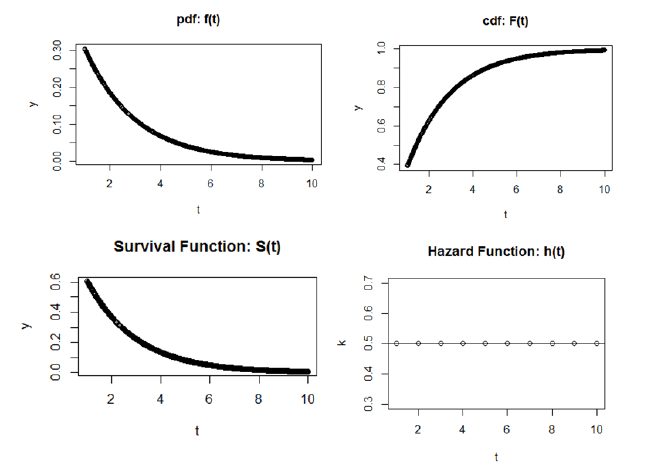If we let h(t) = λ or λ0 or ln h(t) = ln(λ0)  = μ  be the ‘baseline’ hazard,  we can easily extend the model to include covariates as such:

h(t) = λ0(t) exp(β 1X12X2)
ln h(t) = ln[λ0(t) exp(β 1X12X2)]
= ln λ0(t) + ln[exp(β 1X12X2)]
= μ + β 1X12X2
Note: if X1 and X2 = 0 then we get the baseline hazard

Cox Proportional Hazards Model
If we specify the hazard for two individuals i and i’ :

hi(t) = λ0(t) exp(β 1X1i2X2i)
hi’(t) = λ0(t) exp(β 1X1i’2X2i’)

Then the proportional hazard for individual i relative to i’ can be written as:

hi(t)/ hi’(t) = λ0(t) exp(β 1X1i2X2i)/ λ0(t) exp(β 1X1i’2X2i’)
with the ‘baseline’ hazard term cancelling out we get:
hi(t)/ hi’(t) = exp[β 1(X1 i –X1i’)  +β2(X2 i –X2i’) ] or e ζ i- ζ i’

As a result, we don’t have to explicitly know the functional form of the baseline hazard to calculate the proportional hazard.

Partial Likelihood Estimation

The parameters for the proportional hazard model are estimated via partial likelihood estimation.

Introducing a censoring indicator ‘ci’ {=0 if censored at event time else 1} we can express the likelihood function as: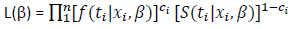Instead of maximizing the full likelihood specified above, Cox proposed estimating the partial likelihood which does not include the baseline hazard function: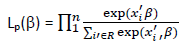The risk set contains subjects at risk for the event at time ‘ti ‘when the event was observed for subject ‘i’. Censoring times are excluded from the likelihood.

Based on the Cox model, hazard was proportional to exp(xi'B) The ratio in the partial likelihood represents the hazard for subject ‘i’ relative to the cumulative hazard for all other subjects at risk at the time subject ‘i’ experienced the event. The estimates for β can be derived by maximizing Lp(β) , and can be interpreted as follows: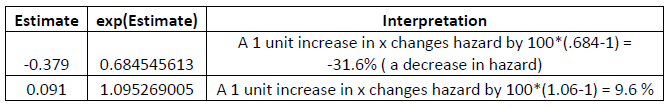References:
Survival Analysis Using SAS: A Practical Guide. Paul D. Allison. 1995. The SAS Institute.
Cox Proportional-Hazards Regression for Survival Data:
Appendix to An R and S-PLUS Companion to Applied Regression. John Fox Februrary 2002

Introduction to Survival Analysis. Sociology 761 Lecture Notes. John Fox. 2006.

Introduction to Survival Analysis. (ppt presentation). Kristin Sainani. Ph.D.  Stanford University. Department of Health Research and Policy. http://www.stanford.edu/~kcobb